Next: The Vector Product Up: Vectors Previous: Vector Area

## The Scalar Product

A scalar quantity is invariant under all possible rotational transformations. The individual components of a vector are not scalars because they change under transformation. Can we form a scalar out of some combination of the components of one, or more, vectors? Suppose that we were to define the ampersand'' product,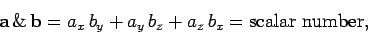(16)

for general vectors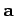and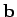. Is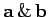invariant under transformation, as must be the case if it is a scalar number? Let us consider an example. Suppose that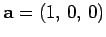and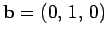. It is easily seen that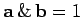. Let us now rotate the basis through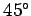about the-axis. In the new basis,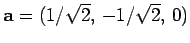and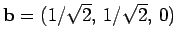, giving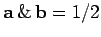. Clearly,is not invariant under rotational transformation, so the above definition is a bad one.

Consider, now, the dot product or scalar product,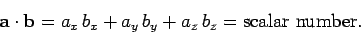(17)

Let us rotate the basis thoughdegrees about the-axis. According to Eqs. (10)-(12), in the new basis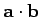takes the form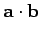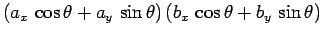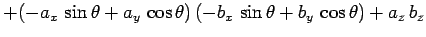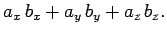(18)

Thus,is invariant under rotation about the-axis. It can easily be shown that it is also invariant under rotation about the- and-axes. Clearly,is a true scalar, so the above definition is a good one. Incidentally,is the only simple combination of the components of two vectors which transforms like a scalar. It is easily shown that the dot product is commutative and distributive: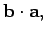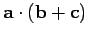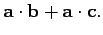(19)

The associative property is meaningless for the dot product, because we cannot have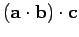, sinceis scalar.

We have shown that the dot productis coordinate independent. But what is the physical significance of this? Consider the special case where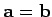. Clearly,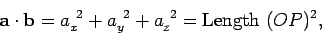(20)

ifis the position vector of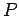relative to the origin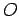. So, the invariance of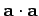is equivalent to the invariance of the length, or magnitude, of vectorunder transformation. The length of vectoris usually denoted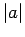(the modulus of'') or sometimes just, so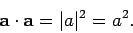(21)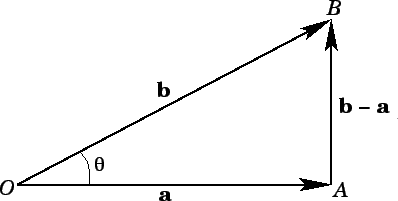Let us now investigate the general case. The length squared ofin Fig. 5 is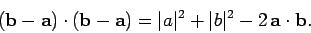(22)

However, according to the cosine rule'' of trigonometry,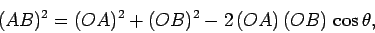(23)

where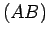denotes the length of side. It follows that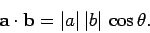(24)

Clearly, the invariance ofunder transformation is equivalent to the invariance of the angle subtended between the two vectors. Note that if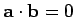then either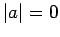,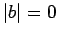, or the vectorsandare mutually perpendicular. The anglesubtended between two vectors can easily be obtained from the dot product: i.e.,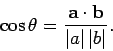(25)

Note that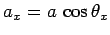, etc., where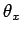is the angle subtended between vectorand the-axis.

The work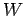performed by a constant force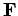which moves an object through a displacement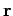is the product of the magnitude oftimes the displacement in the direction of. So, if the angle subtended betweenandisthen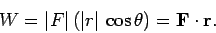(26)Next: The Vector Product Up: Vectors Previous: Vector Area
Richard Fitzpatrick 2007-07-14